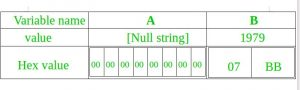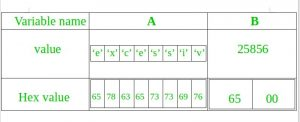# Reasons for a C++ program crash

We sometimes come across abnormal crash of C++ programs. Below are some possible reasons which may cause C++ to crash abnormally.

• Segmentation Fault: It is the major reason for program to crash. These are may be the reasons for the such cause:
• Attempting to access memory location that doesn’t exist in our system.
• There may be an attempt to write on a read only memory location.

 `// CPP program to demonstrate ` `int` `main() ` `{ ` `   ``char` `*str;  ` `  `  `   ``/* Stored in read only part of data segment */` `   ``str = ``"GfG"``;      ` `  `  `   ``/* Problem:  trying to modify read only memory */` `   ``*(str+1) = ``'n'``;  ` `   ``return` `0; ` `} `

Output :

`Segmentation fault (core dumped)`
• There may be an attempt to access protected memory location such as kernel memory
• Stack Overflow: There may case of non terminating recursion with memory location.

 `// C program to demonstrate stack overflow ` `// by creating a non-terminating recursive ` `// function. ` `#include ` ` `  `void` `fun(``int` `x) ` `{ ` `    ``if` `(x == 1) ` `       ``return``; ` `    ``x = 6; ` `    ``fun(x); ` `} ` ` `  `int` `main() ` `{ ` `   ``int` `x = 5; ` `   ``fun(x); ` `} `

Output :

`Segmentation fault (core dumped)`
• Buffer Overflow: It is an anomaly where a program, while writing data to a buffer, overruns the buffer’s boundary and overwrites adjacent memory locations.

• Consider below C++ program.

 `// C++ code to demonstrate buffer ` `// overflow. ` `#include ` `using` `namespace` `std; ` ` `  `// driver code ` `int` `main() ` `{ ` `    ``char` `A = ``""``; ` `    ``unsigned ``short` `B = 1979; ` `    ``strcpy``(A, ``"excessive"``); ` `    ``return` `0; ` `} `

Output :

```*** stack smashing detected ***: /home/gfg/a terminated
Aborted (core dumped)```

The program has two variables which are adjacent in memory: an 8-byte-long string buffer, A, and a two-byte big-endian integer, B.

char A = “”;
unsigned short B = 1979;

Initially, A contains nothing but zero bytes, and B contains the number 1979.Now, the program attempts to store the null-terminated string “excessive” with ASCII encoding in the A buffer.

strcpy(A, “excessive”);

This string is of 9 characters long and encodes to 10 bytes including the null terminator, but A can take only 8 bytes. By failing to check the length of the string, it also overwrites the value of B:B’s value has now been inadvertently replaced by a number formed from part of the character string. In this example “e” followed by a zero byte would become 25856.

This overflow is called buffer buffer overflow.

• Memory Leak:
If we allocate some memory by some program and let it be as it is. After sometime there will be huge memory that is allocated but not used so this would lack of memory after sometime. And thus program start crashing.

 `// C program to demonstrate heap overflow ` `// by continuously allocating memory ` `#include ` `  `  `int` `main() ` `{ ` `    ``for` `(``int` `i=0; i<10000000; i++) ` `    ``{ ` `       ``// Allocating memory without freeing it ` `       ``int` `*ptr = (``int` `*)``malloc``(``sizeof``(``int``)); ` `    ``} ` `} `

• Exceptions

• Divide by zero.

 `// C++ code to demonstrate divide by 0. ` `#include ` `using` `namespace` `std; ` ` `  `// driver code ` `int` `main() ` `{ ` `    ``int` `x = 10; ` `    ``int` `y = 0; ` `    ``cout << x/y; ` `    ``return` `0; ` `}`

Output :

`Floating point exception (core dumped)`

Rated as one of the most sought after skills in the industry, own the basics of coding with our C++ STL Course and master the very concepts by intense problem-solving.

My Personal Notes arrow_drop_upCheck out this Author's contributed articles.

If you like GeeksforGeeks and would like to contribute, you can also write an article using contribute.geeksforgeeks.org or mail your article to contribute@geeksforgeeks.org. See your article appearing on the GeeksforGeeks main page and help other Geeks.

Please Improve this article if you find anything incorrect by clicking on the "Improve Article" button below.

Article Tags :
Practice Tags :

1

Please write to us at contribute@geeksforgeeks.org to report any issue with the above content.